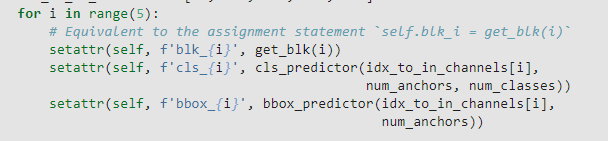# Single Shot Multibox Detection (SSD)

Based on this plot below, I think SSD predicts the classes and bounding boxes of anchor boxes (based on the , so I expect the anchor boxes generated by `multibox_prior` should be explicitly passed into `cls_predictor` and `bbox_predictor`.However, in `blk_forward`, only the feature map `Y` are passed in:

``````def blk_forward(X, blk, size, ratio, cls_predictor, bbox_predictor):
Y = blk(X)
anchors = d2l.multibox_prior(Y, sizes=size, ratios=ratio)
cls_preds = cls_predictor(Y)
bbox_preds = bbox_predictor(Y)
return (Y, anchors, cls_preds, bbox_preds)
``````

So, the SSD model forward propogation outputs classes & labels solely based on feature maps. The anchor boxes are only used during the loss evaluation. Is this statement correct?

Thanks for any correction & thoughts!

in def multibox_prior(data, sizes, ratios):… , sizes should change to size_tensor, this can improve speed!

Hi markchangliu,
the cls_predictor() in function blk_forward, is actually a network, look carefully at class TinySSD:at the forward method:
`for i in range(5):

``````        # Here `getattr(self, 'blk_%d' % i)` accesses `self.blk_i`
X, anchors[i], cls_preds[i], bbox_preds[i] =  blk_forward(
X, getattr(self, f'blk_{i}'), sizes[i], ratios[i],
getattr(self, f'cls_{i}'), getattr(self, f'bbox_{i}'))`
``````

The cls_predictor is actually a network, not the function built in this book, I think you can re-implement it, and then change the name, to make it clearer